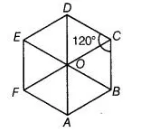# The area of regular hexagon of side`
Question:

The area of regular hexagon of side a is the sum of the areas of the five equilateral triangles with side a.

Solution:

False
We know that regular hexagon is divided into six equilateral triangles.Area of regular hexagon of side a = Sum of area of the six equilateral triangles.